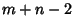## Billiards

The game of billiards is played on a Rectangular table (known as a billiard table) upon which balls are placed. One ball (the ``cue ball'') is then struck with the end of a ``cue'' stick, causing it to bounce into other balls and Reflect off the sides of the table. Real billiards can involve spinning the ball so that it does not travel in a straight Line, but the mathematical study of billiards generally consists of Reflections in which the reflection and incidence angles are the same. However, strange table shapes such as Circles and Ellipses are often considered. Many interesting problems can arise.

Given a rectangular billiard table with only corner pockets and sides of Integer lengthsand(withandRelatively Prime), a ball sent at a 45° angle from a corner will be pocketed in another corner afterbounces (Steinhaus 1983, p. 63).

Alhazen's Billiard Problem seeks to find the point at the edge of a circular ``billiards'' table at which a cue ball at a given point must be aimed in order to carom once off the edge of the table and strike another ball at a second given point. It was not until 1997 that Neumann proved that the problem is insoluble using a Compass and Ruler construction.

On an Elliptical billiard table, the Envelope of a trajectory is a smaller Ellipse, a Hyperbola, a Line through the Foci of the Ellipse, or periodic curve (e.g., Diamond-shape) (Wagon 1991).

References

Davis, D.; Ewing, C.; He, Z.; and Shen, T. ``The Billiards Simulation.'' http://serendip.brynmawr.edu/chaos/home.html.

Dullin, H. R.; Richter, P.H.; and Wittek, A. ``A Two-Parameter Study of the Extent of Chaos in a Billiard System.'' Chaos 6, 43-58, 1996.

Madachy, J. S. ``Bouncing Billiard Balls.'' In Madachy's Mathematical Recreations. New York: Dover, pp. 231-241, 1979.

Neumann, P. Submitted to Amer. Math. Monthly.

Pappas, T. ``Mathematics of the Billiard Table.'' The Joy of Mathematics. San Carlos, CA: Wide World Publ./Tetra, p. 43, 1989.

Peterson, I. ``Billiards in the Round.'' http://www.sciencenews.org/sn_arc97/3_1_97/mathland.htm.

Steinhaus, H. Mathematical Snapshots, 3rd American ed. New York: Oxford University Press, 1983.

Wagon, S. ``Billiard Paths on Elliptical Tables.'' §10.2 in Mathematica in Action. New York: W. H. Freeman, pp. 330-333, 1991.